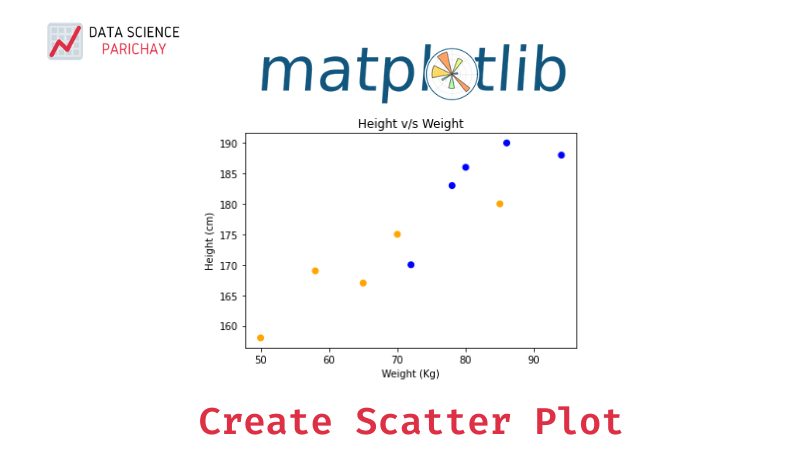# Create a Scatter Plot in Python with Matplotlib

Scatter plots are great for visualizing data points in two dimensions. They’re particularly useful for showing correlations and groupings in data. In this tutorial, we’ll look at how to create a scatter plot in python using matplotlib.

Matplotlib is a library in python used for visualizing data. It offers a range of different plots and customizations. In matplotlib, you can create a scatter plot using the pyplot’s `scatter()` function. The following is the syntax:

``````import matplotlib.pyplot as plt
plt.scatter(x_values, y_values)``````

Here, `x_values` are the values to be plotted on the x-axis and `y_values` are the values to be plotted on the y-axis.

Let’s look at some of the examples of plotting a scatter diagram with matplotlib.

We have the data for heights and weights of 10 students at a university and want to plot a scatter plot of the distribution between them. The data is present in two lists. One having the height and the other having the corresponding weights of each student.

``````import matplotlib.pyplot as plt

# height and weight data
height = [167, 175, 170, 186, 190, 188, 158, 169, 183, 180]
weight = [65, 70, 72, 80, 86, 94, 50, 58, 78, 85]

# plot a scatter plot
plt.scatter(weight, height)
plt.show()``````

Output:

We get a scatter chart with data points plotted on a chart with weights on the x-axis and heights on the y-axis. From the chart, we can see that there’s a positive correlation in the data between height and weight.

The scatter plot that we got in the previous example was very simple without any formatting. Matplotlib comes with number of different formatting options to customize your charts. Let’s add some formatting to the above chart.

📚 Data Science Programs By Skill Level

Introductory

Intermediate ⭐⭐⭐

🔎 Find Data Science Programs 👨‍💻 111,889 already enrolled

Disclaimer: Data Science Parichay is reader supported. When you purchase a course through a link on this site, we may earn a small commission at no additional cost to you. Earned commissions help support this website and its team of writers.

Matplotlib’s pyplot has handy functions to add axis labels and title to your chart. Let’s add them to the chart created above:

``````import matplotlib.pyplot as plt

# height and weight data
height = [167, 175, 170, 186, 190, 188, 158, 169, 183, 180]
weight = [65, 70, 72, 80, 86, 94, 50, 58, 78, 85]

# plot a scatter plot
plt.scatter(weight, height)
# set axis lables
plt.xlabel("Weight (Kg)")
plt.ylabel("Height (cm)")
# set chart title
plt.title("Height v/s Weight")
plt.show()``````

Output:

The scatter plots above have round markers. You can alter the shape of the marker with the `marker` parameter and size of the marker with the `s` parameter of the `scatter()` function. For instance, to make the markers start-shaped instead of the round with larger size:

``````import matplotlib.pyplot as plt

# height and weight data
height = [167, 175, 170, 186, 190, 188, 158, 169, 183, 180]
weight = [65, 70, 72, 80, 86, 94, 50, 58, 78, 85]

# plot a scatter plot with star markers
plt.scatter(weight, height, marker='*', s=80)
# set axis lables
plt.xlabel("Weight (Kg)")
plt.ylabel("Height (cm)")
# set chart title
plt.title("Height v/s Weight")
plt.show()``````

Output:

You can also have different colors for different data points in matplotlib’s scatter plot. This is very useful if your data points belonging to different categories. For instance, in the above example, if we add data corresponding to the nationalities of the students say country A and B and want to display each country with a different color:

``````import matplotlib.pyplot as plt

# height, weight and country data
height = [167, 175, 170, 186, 190, 188, 158, 169, 183, 180]
weight = [65, 70, 72, 80, 86, 94, 50, 58, 78, 85]
country = ['A', 'A', 'B', 'B', 'B', 'B', 'A', 'A', 'B', 'A']

# color map for each category
colors = {'A':'orange', 'B':'blue'}
color_ls = [colors[i] for i in country]

# plot
plt.scatter(weight, height, c=color_ls)
plt.xlabel("Weight (Kg)")
plt.ylabel("Height (cm)")
plt.title("Height v/s Weight")
plt.show()``````

Output:

You can see that data points for A are colored orange while data points for B are blue. This gives another insight that students from country A tend to have lower height and weight than students from B based on the given data.

For more on the maplotlib scatter plot function, refer to its documentation.

With this, we come to the end of this tutorial. The code examples and results presented in this tutorial have been implemented in a Jupyter Notebook with a python (version 3.8.3) kernel having matplotlib version 3.2.2

•